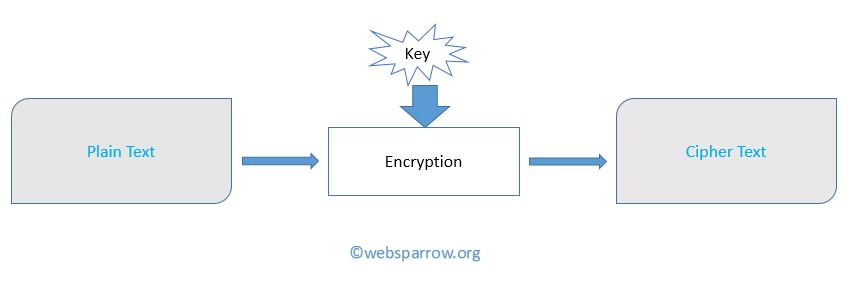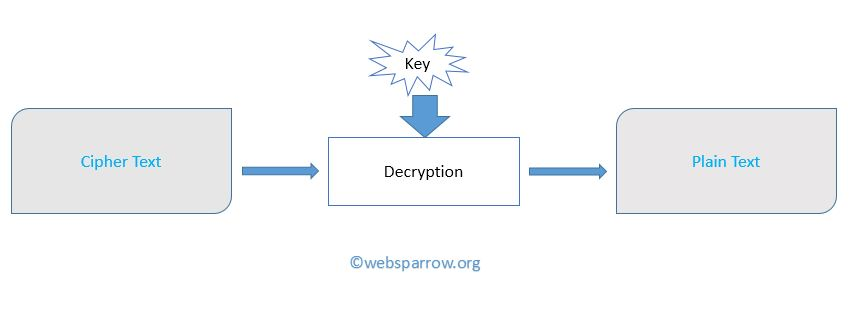# How to Encrypt and Decrypt data in Java

In this tutorial we are going to explain how to `Encrypt` and `Decrypt` plain text data in a simple way. In this example, we are going to use a simple `Key` to encrypt the data and the same `Key` to decrypt the encrypted data.

`3` is used as a key to encrypt and decrypt the data.

### What is Encryption?

Encryption is a mechanism of encoding the data in such a way that only authorized member can access it. Encode data is called Cipher Text.### What is Decryption?

Decryption is a technique in which we decrypt the encrypted data using same `Key` that is used while encrypting the data.Note: In this example we are using the same `Key` to encrypt and decrypt the data. When the encryption and decryption keys are the same this is called Symmetric key / Private key.

PlainText.txt
``WebSparrow.org is an Internet company. It will provide high quality and well tested technical pages for Internet techie.``

### Encryption Example

In this example, we are going to read the data from PlainText.txt file and apply the encryption key and write the encrypted data in CipherText.txt file.

EncryptionExp.java
``````package org.websparrow;

import java.io.FileInputStream;
import java.io.FileOutputStream;

public class EncryptionExp {
public static void main(String[] args) {
try {

int ctr;

FileInputStream inputStream = new FileInputStream("PlainText.txt");
FileOutputStream outputStream = new FileOutputStream("CipherText.txt");

while ((ctr = inputStream.read()) != -1) {
ctr -= 3; // 3 is the encryption key .
System.out.print((char) ctr);
outputStream.write(ctr);
}
outputStream.close();
} catch (Exception ex) {
ex.printStackTrace();
}
}
}``````

Output:

Finally, plain text has been encoded as given below…

``Tb_Pm^oolt+lodfp^kFkqbokbq`ljm^kv+Fqtfiimolsfabefdenr^ifqv^katbiiqbpqbaqb`ekf`^im^dbpcloFkqbokbqqb`efb+``

### Decryption Example

This example decrypt the above-encrypted data and write it into OriginalText.txt

DecryptionExp.java
``````package org.websparrow;

import java.io.FileInputStream;
import java.io.FileOutputStream;

class DecryptionExp {
public static void main(String[] args) {
try {

int ctr;

FileInputStream inputStream = new FileInputStream("CipherText.txt");
FileOutputStream outputStream = new FileOutputStream("OriginalText.txt");

while ((ctr = inputStream.read()) != -1) {

ctr += 3; // 3 is the decryption key.

System.out.print((char) ctr);
outputStream.write(ctr);
``WebSparrow.org is an Internet company. It will provide high quality and well tested technical pages for Internet techie.``